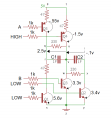# calculating voltages between nodes of a circuit

#### mikewax

Joined Apr 11, 2016
170Hello,
here is a picture of the voltages across the different components in my circuit. The purpose is to charge a pair of supercaps one at a time, first C1 then C2. At this point in the picture, C1 has just finished charging and i'm measuring the various voltages.
the sequence goes as follows:

initial state: V$$_{C1}$$ = V$$_{C2}$$ = 0
transistors Q1, Q2, and Q4 remain in the cutoff state.
1- A = LOW, B = HIGH (begin charging C1, current goes from p->r->v->z )
2- when C1 reaches 2.5v, A goes HIGH and B goes LOW (all Qs now in cutoff).

all voltage readings are made roughly at the moment of cutoff as C1 starts to discharge.
can someone help me with the arithmetic? i can't figure out these voltage readings.
thanx, louie

Last edited:

#### ci139

Joined Jul 11, 2016
1,696
at the time i figured out the wave form diagram for the symmetrical astable multivibrator only knowing the Ohm's Law and some basics of bjt - without computing anything just simulating the circuit in my mind - i was amazed to confirm with the multimeter and a diode the negative voltages developed at the bases (it was before the internet era, i didn't own a PC then until few years later (and i was one of few in my country to have 1 then) - at secondary school time)

so basically draw out the circuit wave forms - divide/group it to a different cycles operation - just exacten/correct the voltage values using www for any formulas needed